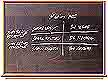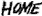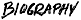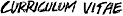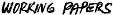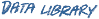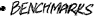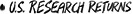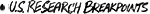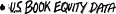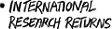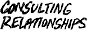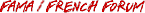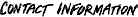Variable Definitions

 ME Market Equity. Market equity (size) is price times shares outstanding. Price is from CRSP, shares outstanding are from Compustat (if available) or CRSP. BE Book Equity. Book equity is constructed from Compustat data or collected from the Moodys Industrial, Financial, and Utilities manuals. BE is the book value of stockholders equity, plus balance sheet deferred taxes and investment tax credit (if available), minus the book value of preferred stock. Depending on availability, we use the redemption, liquidation, or par value (in that order) to estimate the book value of preferred stock. Stockholders equity is the value reported by Moodys or Compustat, if it is available. If not, we measure stockholders equity as the book value of common equity plus the par value of preferred stock, or the book value of assets minus total liabilities (in that order). See Davis, Fama, and French, 2000, Characteristics, Covariances, and Average Returns: 1929-1997, Journal of Finance, for more details. BE/ME Book-to-Market. The book-to-market ratio used to form portfolios in June of year t is book equity for the fiscal year ending in calendar year t-1, divided by market equity at the end of December of t-1. OP Operating Profitability. The operating profitability ratio used to form portfolios in June of year t is annual revenues minus cost of goods sold, interest expense, and selling, general, and administrative expense divided by the sum of book equity and minority interest for the last fiscal year ending in t-1. INV Investment. The investment ratio used to form portfolios in June of year t is the change in total assets from the fiscal year ending in year t-2 to the fiscal year ending in t-1, divided by t-2 total assets. E/P Earnings/Price. Earnings is total earnings before extraordinary items, from Compustat. The earnings/price ratio used to form portfolios in June of year t is earnings for the fiscal year ending in calendar year t-1, divided by market equity at the end of December of t-1. CF/P Cashflow/Price. Cashflow is total earnings before extraordinary items, plus equitys share of depreciation, plus deferred taxes (if available), from Compustat. Equitys share is defined as market equity divided by assets minus book equity plus market equity. The cashflow/price ratio used to form portfolios in June of year t is the cashflow for the fiscal year ending in calendar year t-1, divided by market equity at the end of December of t-1. D/P Dividend Yield. The dividend yield used to form portfolios in June of year t is the total dividends paid from July of t-1 to June of t per dollar of equity in June of t. The dividend yield is computed using the with and without dividend returns from CRSP, as described in Fama and French, 1988, Dividend yields and expected stock returns, Journal of Financial Economics 25.

Copyright Kenneth R. French